Worksheets and No Prep Teaching Resources
Math Worksheets

# Number Lines Worksheets

Number lines help students make sense of addition and subtraction and help students develop a visual reference for these important skills. Number lines help students accelerate their mental math abilities, too. These practical worksheets will be a great tool for students to understand number lines and use them to accelerate their skills!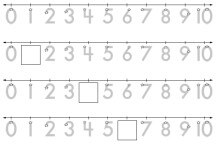Easy Number Lines - Trace Font - No blanks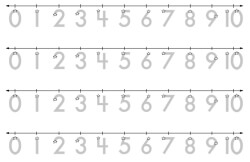Printable math number Line from 0 to 5 - no blanks Printable math number Line from 0 to 9 - no blanks Printable math number Line from 0 to 10 - no blanks Printable math number Line from 1 to 9 - no blanks Printable math number Line from 1 to 10 - no blanks

Easy Number Lines - Trace Font - 1 Blank / First Number Line has No Blanks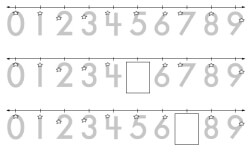Printable math number Line from 0 to 5 - (1 blank after first number line) Printable math number Line from 0 to 9 - (1 blank after first number line) Printable math number Line from 0 to 10 - (1 blank after first number line) Printable math number Line from 1 to 9 - (1 blank after first number line) Printable math number Line from 1 to 10 - (1 blank after first number line)

Traceable Number Lines - More Blanks

Number Lines - Positive Numbers - Blanks After First Number Line

Number Lines - Positive Numbers - Each Number Line Starts at DIFFERENT Number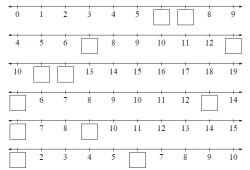5 Numbers on Number Line - Printable math number Line from 0 to 10 - (1 blank) 5 Numbers on Number Line - Printable math number Line from 0 to 10 - (2 blanks) 10 Numbers on Number Line - Printable math number Line from 0 to 25 - (2 blanks)

Number Lines - Negatives Numbers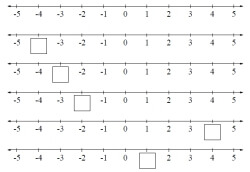Printable math number Line from -5 to 5 - (1 blank after first number line) Printable math number Line from -10 to 10 - (1 blank after first number line) Printable math number Line from -5 to 5 - (3-5 blanks after first number line) Printable math number Line from -10 to 10 - (3-5 blanks after first number line)

Number Lines - Negatives Numbers - Each Number Line Starts at DIFFERENT Number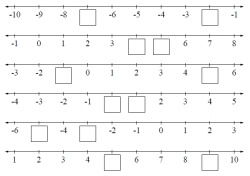5 Numbers on Number Line - Printable math number Line from -5 to 5 - (1 blank) 5 Numbers on Number Line - Printable math number Line from -5 to 5 - (2 blanks) 5 Numbers on Number Line - Printable math number Line from -10 to 10 - (1 blank) 5 Numbers on Number Line - Printable math number Line from -10 to 10 - (2 blanks) 10 Numbers on Number Line - Printable math number Line from -10 to 10 - (2 blanks)

Fraction Number Lines

Place Value Number Lines
Ones and Tens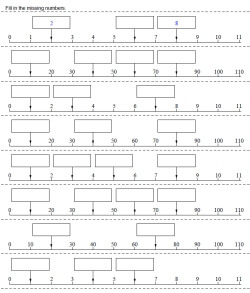Ones and tens number line - all start from 0 (couple answers given) Ones and tens number line - all start from 0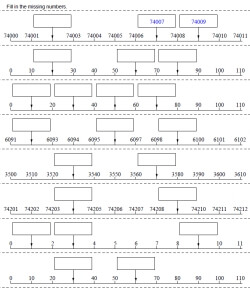Ones and tens number line - half of the problems start from 0 (couple answers given) Ones and tens number line - half of the problems start from 0 Ones and tens number line (couple answers given) Ones and tens number line

Ones, Tens, and Hundreds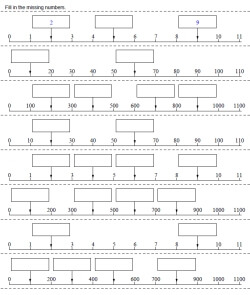Ones, tens, and hundreds number line - all start from 0 (couple answers given) Ones, tens, and hundreds number line - all start from 0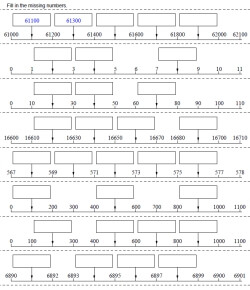Ones, tens, and hundreds number line - half of the problems start from 0 (couple answers given) Ones, tens, and hundreds number line - half of the problems start from 0 Ones, tens, and hundreds number line (couple answers given) Ones, tens, and hundreds number line

Ones, Tens, Hundreds, and Thousands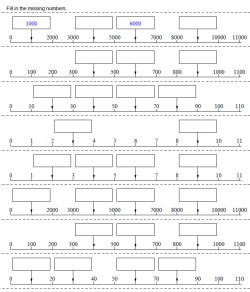Ones, tens, hundreds, and thousands number line - all start from 0 (couple answers given) Ones, tens, hundreds, and thousands number line - all start from 0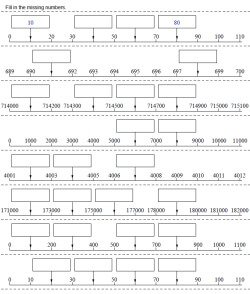Ones, tens, hundreds, and thousands number line - half of the problems start from 0 (couple answers given) Ones, tens, hundreds, and thousands number line - half of the problems start from 0 Ones, tens, hundreds, and thousands number line (couple answers given) Ones, tens, hundreds, and thousands number line